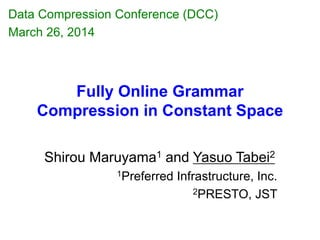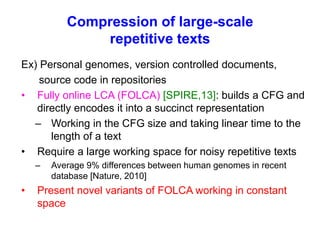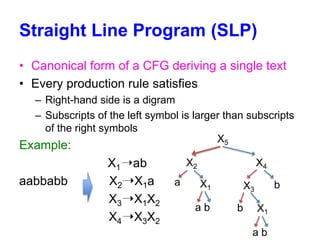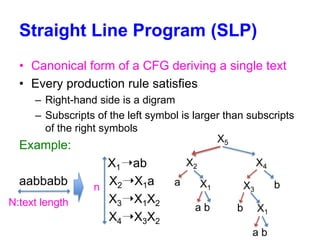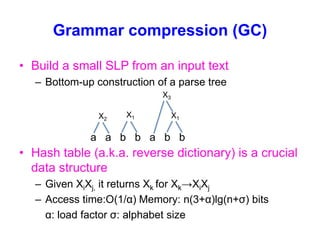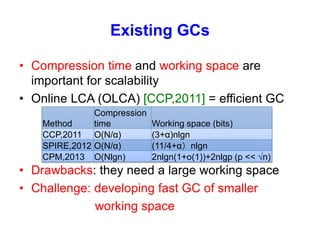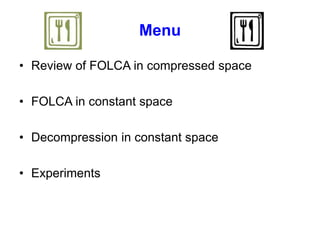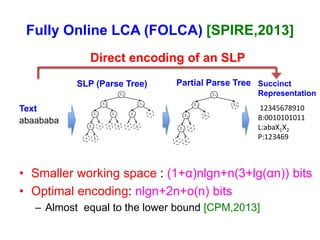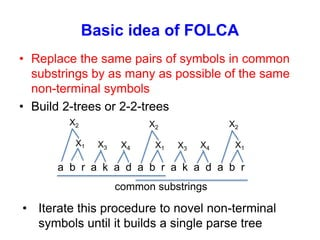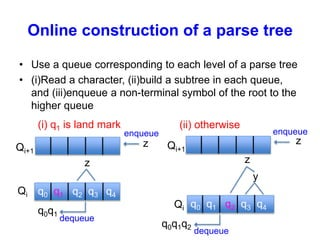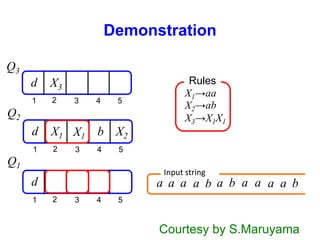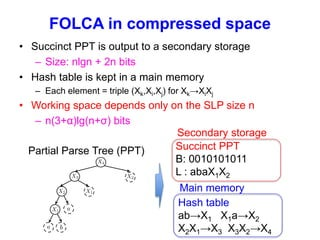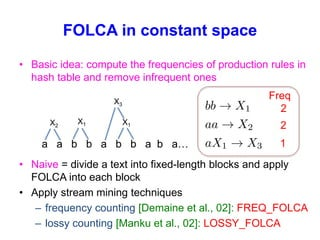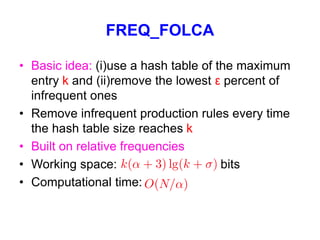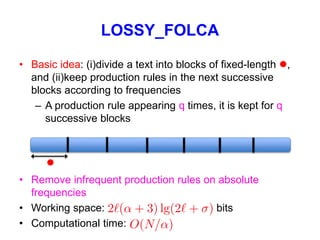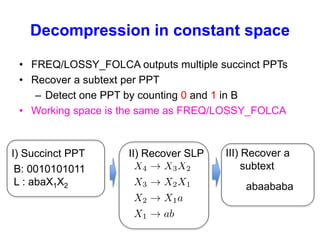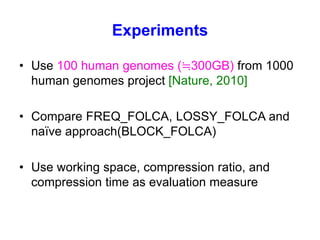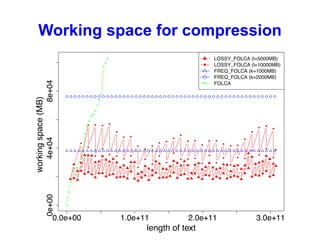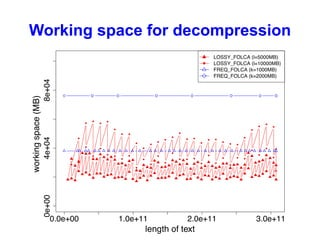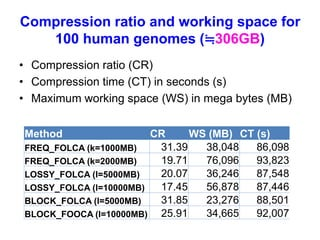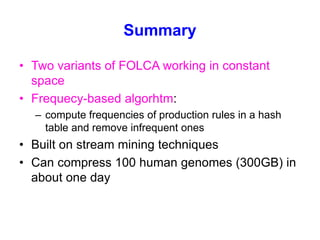1 of 21

### DCC2014 - Fully Online Grammar Compression in Constant Space

1. Fully Online Grammar Compression in Constant Space Shirou Maruyama1 and Yasuo Tabei2 1Preferred Infrastructure, Inc. 2PRESTO, JST Data Compression Conference (DCC) March 26, 2014
2. Compression of large-scale repetitive texts Ex) Personal genomes, version controlled documents, source code in repositories • Fully online LCA (FOLCA) [SPIRE,13]: builds a CFG and directly encodes it into a succinct representation – Working in the CFG size and taking linear time to the length of a text • Require a large working space for noisy repetitive texts – Average 9% differences between human genomes in recent database [Nature, 2010] • Present novel variants of FOLCA working in constant space
3. Straight Line Program (SLP) • Canonical form of a CFG deriving a single text • Every production rule satisfies – Right-hand side is a digram – Subscripts of the left symbol is larger than subscripts of the right symbols Example: X1➝ab aabbabb X2➝X1a X3➝X1X2 X4➝X3X2 a b a X2 X1 X1 X3 X4 X5 b b a b
4. Straight Line Program (SLP) • Canonical form of a CFG deriving a single text • Every production rule satisfies – Right-hand side is a digram – Subscripts of the left symbol is larger than subscripts of the right symbols Example: X1➝ab aabbabb X2➝X1a X3➝X1X2 X4➝X3X2 a b a X2 X1 X1 X3 X4 X5 b b a b n N:text length
5. Grammar compression (GC) • Build a small SLP from an input text – Bottom-up construction of a parse tree • Hash table (a.k.a. reverse dictionary) is a crucial data structure – Given XiXj, it returns Xk for Xk→XiXj – Access time:O(1/α) Memory: n(3+α)lg(n+σ) bits α: load factor σ: alphabet size a a b b a b b X1 X1X2 X3
6. Existing GCs • Compression time and working space are important for scalability • Online LCA (OLCA) [CCP,2011] = efficient GC • Drawbacks: they need a large working space • Challenge: developing fast GC of smaller working space Method Compression time Working space (bits) CCP,2011 O(N/α) (3+α)nlgn SPIRE,2012 O(N/α) (11/4+α）nlgn CPM,2013 O(Nlgn) 2nlgn(1+o(1))+2nlgp (p << √n)
7. Menu • Review of FOLCA in compressed space • FOLCA in constant space • Decompression in constant space • Experiments
8. Fully Online LCA (FOLCA) [SPIRE,2013] • Smaller working space : (1+α)nlgn+n(3+lg(αn)) bits • Optimal encoding: nlgn+2n+o(n) bits – Almost equal to the lower bound [CPM,2013] abaababa 12345678910 B:0010101011 L:abaX1X2 P:123469 Text SLP (Parse Tree) Partial Parse Tree Succinct Representation Direct encoding of an SLP
9. Basic idea of FOLCA • Replace the same pairs of symbols in common substrings by as many as possible of the same non-terminal symbols • Build 2-trees or 2-2-trees a b r a k a d a b r a k a d a b r common substrings X1 X2 X1 X2 X4 X1 X2 X3 X3 X4 • Iterate this procedure to novel non-terminal symbols until it builds a single parse tree
10. Online construction of a parse tree • Use a queue corresponding to each level of a parse tree • (i)Read a character, (ii)build a subtree in each queue, and (iii)enqueue a non-terminal symbol of the root to the higher queue Qi q0 q1 q2 q3 q4 z zQi+1 enqueue dequeue q0q1 Qi q0 q1 q2 q3 q4 zQi+1 enqueue dequeue q0q1q2 y z (i) q1 is land mark (ii) otherwise
11. Demonstration 1 2 3 4 5 d 1 2 3 4 5 d 1 2 3 4 5 d Q1 Q2 Q3 aaa X1→aa X1 a abab a a a b X1 X2→ab b X2 X3→X1X1 X3 Rules Input string Courtesy by S.Maruyama
12. FOLCA in compressed space • Succinct PPT is output to a secondary storage – Size: nlgn + 2n bits • Hash table is kept in a main memory – Each element = triple (Xk,Xi,Xj) for Xk→XiXj • Working space depends only on the SLP size n – n(3+α)lg(n+σ) bits Partial Parse Tree (PPT) Succinct PPT B: 0010101011 L : abaX1X2 Secondary storage Hash table ab→X1 X1a→X2 X2X1→X3 X3X2→X4 Main memory
13. FOLCA in constant space • Basic idea: compute the frequencies of production rules in hash table and remove infrequent ones • Naive = divide a text into fixed-length blocks and apply FOLCA into each block • Apply stream mining techniques – frequency counting [Demaine et al., 02]: FREQ_FOLCA – lossy counting [Manku et al., 02]: LOSSY_FOLCA a a b b a b b a b a… X1 X1X2 X3 Freq 2 2 1
14. FREQ_FOLCA • Basic idea: (i)use a hash table of the maximum entry k and (ii)remove the lowest ε percent of infrequent ones • Remove infrequent production rules every time the hash table size reaches k • Built on relative frequencies • Working space: bits • Computational time:
15. LOSSY_FOLCA • Basic idea: (i)divide a text into blocks of fixed-length l, and (ii)keep production rules in the next successive blocks according to frequencies – A production rule appearing q times, it is kept for q successive blocks • Remove infrequent production rules on absolute frequencies • Working space: bits • Computational time: l
16. Decompression in constant space • FREQ/LOSSY_FOLCA outputs multiple succinct PPTs • Recover a subtext per PPT – Detect one PPT by counting 0 and 1 in B • Working space is the same as FREQ/LOSSY_FOLCA B: 0010101011 L : abaX1X2 abaababa I) Succinct PPT II) Recover SLP III) Recover a subtext
17. Experiments • Use 100 human genomes (≒300GB) from 1000 human genomes project [Nature, 2010] • Compare FREQ_FOLCA, LOSSY_FOLCA and naïve approach(BLOCK_FOLCA) • Use working space, compression ratio, and compression time as evaluation measure
18. Working space for compression
19. Working space for decompression
20. Compression ratio and working space for 100 human genomes (≒306GB) • Compression ratio (CR) • Compression time (CT) in seconds (s) • Maximum working space (WS) in mega bytes (MB) Method CR WS (MB) CT (s) FREQ_FOLCA (k=1000MB) 31.39 38,048 86,098 FREQ_FOLCA (k=2000MB) 19.71 76,096 93,823 LOSSY_FOLCA (l=5000MB) 20.07 36,246 87,548 LOSSY_FOLCA (l=10000MB) 17.45 56,878 87,446 BLOCK_FOLCA (l=5000MB) 31.85 23,276 88,501 BLOCK_FOOCA (l=10000MB) 25.91 34,665 92,007
21. Summary • Two variants of FOLCA working in constant space • Frequecy-based algorhtm: – compute frequencies of production rules in a hash table and remove infrequent ones • Built on stream mining techniques • Can compress 100 human genomes (300GB) in about one day

### Editor's Notes

1. In this talk, I will deal with compression of large-scale repetitive texts. Examples are personal genomes, version controlled documents, source code in repositories. We presented fully online LCA called FOLCA that builds an SLP and directly encodes it into a succinct representation. Working space is the SLP size and computational time is linear to the length of a text However, recent sequencing technology generates noisy repetitive texts. Actually, there is 9% difference on average between human genomes in recent database, qlthough it is said that the difference between individual genomes is 0.01%. For such noisy repetitve texts, FOLCA working in the SLP size consumes a large amount of memory. We present novel variants of FOLCA working in constant space.
2. In this talk, we assumes straight line programs for grammars. SLP is a canonical form of a CFG deriving a single string. Every production rule satisfies: right-hand side is a digram Subscripts of the left symbol is larger than subscripts of the right symbols.
3. Grammar compression (GC) builds a small SLP from an input text. It builds a parse tree corresponding to an SLP in a bottom-up manner. Hash table also known as reverse dictionary is a crucial data structure in grammar compressions. Given right hand side of symbols XiXj, it return the right symbol Xk in a production rule Xk ¥to XiXj Access time is O(1/alpha), memory is n(3+alpha)lg(n+alpha) bits Alpha: load factor
4. Compression time and working space are important for applying grammar compression for large-scale repetitive texts. Online LCA (OLCA) is an efficient grammar compression. OLCA is extend as achieving a smaller working space. But, they still need a large working space. Now our challenge is to develop fast GC of smaller working space.
5. We modify FOLCA as working in compressed space. FOLCA builds POPPT that is output to a secondary storage device. The succinct representation is indexed by a rank/select dictionary. There is no small O(n)　here. In addition, hash table is kept in a main memory. The hash table consumes most of the memory. Working space is n(3+alpha)lg(n+sigma) bits. Thus, the working space depends only on the SLP size n.
6. From this slide, I will present FOLCA working in constant space. Basic idea of our novel variants of FOLCA is to compute the frequencies of production rules in hash table and remove infrequent ones at a point We apply stream mining techniques in data mining area for extracting frequent items in data streams. We apply two techniques. First is frequency counting proposed by Demaine et al 2002. We shall referrer to FOLCA using frequency counting as FREQ_FOLCA. Second is lossy counting proposed by Manku et al in 2002. We shall referrer to FOLCA using lossy counting as LOSSY_FOLCA. Naïve approach to compress long repetitive texts is to divide a text into fixed-length blocks and apply compressors into each block. Compression is ruined because long range repetitions are not captured. On the otherhand, our variants of FOLCA can capture long range repetitions.
7. Basic idea of FREQ_FOLCA is to use a hash table of the maximum entry k and remove the lowest ε percent of infrequent one.
8. Basic idea of LOSSY_
9. First figure shows that working space by increasing the length of text. The horizontal axis represents the length of texts. The vertical axis represents working space in megabytes. We tried two parapeters for LOSSY_FOLCA and FREQ_FOLCA. The working space of FOLCA is increasing for the long input texts. FOLCA works in the SLP size. It is not applicable to large-scale, noisy repetitive texts. On the otherhan, our method, LOSSY_FOLCA and FREQ_FOLCA works in the constant space that does not depend on the text length.
10. Second figure shows that the working space for decompression. The horizontal axis represents the length of texts. The vertical axis represents the working space in megabytes. You can see the same trends in the working space for decompression as in that for compression. The working space for LOSSY_FOLCA and FREQ_FOLCA remains constant not depending on the length of text.
11. The last figure shows that compression ratio and working space for 100 human genomes. Compression fished for about one day. You can see the trade off between compression ratio and working space for each method. The larger value of parameters achieves high compression ratio. There are trade off between compression ratio and working space. The compression ratio of LOSSY_FOLCA is better than that of BLOCK_FOLCA for the same block length, which showed that the strategy of LOSSY_FOLCA for removing infrequent production rules was more effective that that of BLOCK_FOLCA. LOSSY_FOLCA using a smaller working space achieved a high compression ratio that FREQ_FOLCA. These results demostrate that applicalities of our method to large-scale repetitive texts.